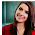# Computer System Architecture Set 34

### Questions 331 to 340

 331 (x’)’ is ________________                                                                                                              (a)    x2                            (b) 0                                (c) 1                                (d) x                                (e) x’. 332 A combinational circuit that converts binary information from n- input lines to a maximum of 2n unique output lines is __________________ (a)    Multiplexer           (b) Full adder                (c) Half adder               (d) Encoder                   (e) Decoder. 333 What does D stand for in D-flip flop? (a)    Direct                     (b) Don’t care               (c) Data                          (d) Double                     (e) Delay. 334 The binary cell capable of storing one bit of information is called ______ (a)    Combination circuit                                    (b) Sequential circuit   (c) Clock                        (d) Latch                                                                (e) Flip flop. 335 ‘n’ number of bits gives _________________ number of combinations in a binary system. (a)    2n                           (b) 2n                               (c) 2n                               (d) 2+n                           (e) 2-n. 336 The performance of the cache memory is measured in terms of a quantity called ___________ (a)    Initialization Ratio                                      (b) Address Ratio                                                 (c) Hit Ratio                                (d) Instruction Ratio    (e) Miss Ratio. 337 Which of the following is an example of sequential access media? (a)    Main Memory                                              (b) Cache memory      (c) Magnetic disk (d) Magnetic tape                                                 (e) CD. 338 The expanded form of ASCII is _________________ (a)    American Standard Code for Information Inventory (b)    American Standard Code for Information Interchange (c)    American Standard Code for Interchange Information (d)    American Standard Code for Inventory Interchange (e)    American Standard Code for Interchange Inventory. 339 Floating point is always interpreted to represent a number in the following form. (a)    m * re                     (b) me * r                        (c) er * m                        (d) m * e                        (e) em * r. 340 _______________ is the number system understood by the computer. (a)    Octal                      (b) Hexa decimal         (c) Decimal                    (d) Binary                      (e) Assembly.

 331 Answer :   (d) Reason :   The complement of x’ is x itself 332 Answer :   (e) Reason :   A combinational circuit that converts binary information from n- input lines to a maximum of 2n unique output lines is Decoder 333 Answer :   (c) Reason :   The D stands for Data. 334 Answer :   (e) Reason :   The binary cell capable of storing one bit of information is called Flip flop. 335 Answer :   (c) Reason :   ‘n’ number of bits gives 2n number of combinations in a binary system. 336 Answer :   (c) Reason :   The performance of the cache memory is measured in terms of a quantity called Hit ratio 337 Answer :   (d) Reason :   Example of sequential access media is Magnetic tape 338 Answer :   (b) Reason :   Acronym for ASCII is American Standard Code for Information Interchange 339 Answer :   (a) Reason :   Floating point is always interpreted to represent a number as m * re 340 Answer :   (d) Reason :   Binary is the number system understood by the computer.

29  30  31  32  33  34  35  36  37  38  39  Next >>

1.2.